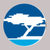cancel
Showing results for
Did you mean:

# PSoC™ 4New Contributor II

## Amux resistance

Hello,

I'm using the PSOC 4200M.  I designed a circuit using an opamp in non-inverting mode.  The Gain resistors are off chip.  I noticed that as I increase the resistors past a gain of one, the gain appears to increase in a non-linear fashion.  I'm using large value resistors to keep the current low.  I am also using an Analog mux to switch in the feedback resistors.

My guess is the internal resistance to the AMUX is a function of current. Here are the resistor values I'm using:

Rin (negative terminal) = 1 M Ohm

Feedback resistors switched in (one at a time) =  1 M ohm,  2.49 M ohms, 7.2 Mohms

These values equate to gains of 2, 3.49 and 8.2.  However, the actual gains calculated from measuring the input and output voltages of the opamp are 2, 4.38 and 11.59 respectively.

Is there a function that describes this behavior?

thanks

Shawn

1 SolutionHonored Contributor II

## Re: Amux resistance

Shawn,

Take a look at this thread,

Re: Trying to configure the OpAmp for Mode:OpAmp and Mode:Follower at run-time

For PSoC5LP Opamps are low impedance. PSoC 4 is likely to be similar. Try to use resistor divider of lower values (/10) .

/odissey1

5 RepliesModerator

## Re: Amux resistance

Hello Shawn,

Can you kindly describe the method you have used to compute the gain by measuring input and output voltages of the opamp?

Best regards,

Sampath SelvarajNew Contributor II

## Re: Amux resistance

To calculate the gain, I have constructed voltage followers using available opamps and send the output of these followers to output pins. The inputs are tied to the input and output of the main opamp.Honored Contributor II

## Re: Amux resistance

Shawn,

I believe that PSoC Opamp has low Input impedance of about 1M, which is comparable to your external resistor values, resulting in higher gain than calculated one by standard equation (1+R2/R1). The AMUX impedance should be under 1k, probably unimportant here.

/odissey1New Contributor II

## Re: Amux resistance

That is a very low input resistance. Can you confirm if this is the case?

Have any other users complained of gain issues with opamps? Is there a best practices guide for setting up and using internal opamps?

Thank youHonored Contributor II

## Re: Amux resistance